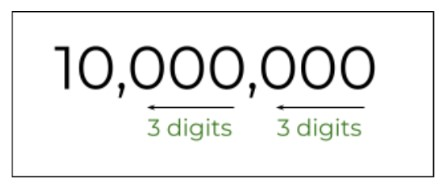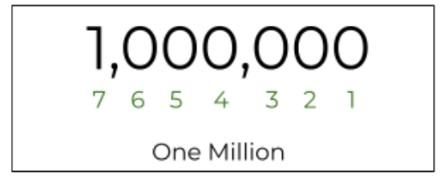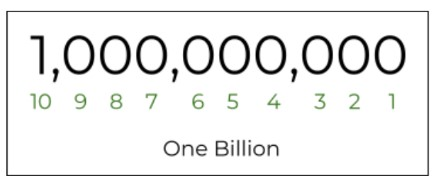# Million to Billion & Billion to Million Converter

To convert million to billion or billion to million, enter value & click the calculate button

Give Us Feedback

## Million to Billion Converter

Million to billion and billion to million converter is an online calculator to change one place value number from the International numbering system to another.

It also gives the conversion of numbers into different place values from the Indian numbering system e.g. lakhs and crores.

## What is the million to billion conversion?

The conversion of millions to billions means the representation of a small number, value, and amount in terms of a higher place value.

Both million and billion are the place values in the international or Western numbering system. In this system, a comma is placed after every three digits from the right.### What is a million?

A value containing 7 digits or six zeros after one is represented by the word million in the number system.### What is a billion?

It is bigger than a million and comes after 100 million in the place value system.## How to convert millions to billions?

In the international number system, after every 3 digits from a hundred, a new term is introduced. First thousand then million and after million comes billion.

Therefore, each higher place value has 1000 (thousand) smaller place values. This is also the case with billions and millions. One billion has 1000 million in it as they come side by side.

To convert from millions to billions, the formula is:

= Number / 1000

To convert from billions to millions, the formula is:

= Number x 1000

Example:

How many billions are in 945 million?

Solution:

`One million = One billion / 1000`
= 0.001 billion

945 millions = 945 x 0.001 billion
= 0.945 billion

## Conversion tables:

### Million to billion:

 Value in Million Value in Billion 1 Million 0.001 Billion 5 Million 0.005 Billion 10 Million 0.01 Billion 100 Million 0.1 Billion 1,000 Million 1 Billion 16.9 Million 0.0169 Billion 34/20 Million 0.0017 Billion 5,000 Million 5 Billion 10,000 Million 10 Billion 100,000 Million 100 Billion

### Billion to million:

 Value in Billion Value in Million 1 Billion 1,000 Million 5 Billion 5,000 Million 0.01 Billion 10 Million 0.001 Billion 1 Million 50 Billion 50,000 Million 23 billion 23,000 Million 1,000 Billion 1,000,000 Million 100 Billion 100,000 Million 1.3 Billion 1,300 Million 2.7 Billion 2,700 Million

### Math Tools